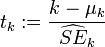A t statistic is calculated any time you do the following: Take a sample statistic that is assumed to be normally distributed. Subtract its expected value from it (e.g., the value predicted by the null hypothesis). Divide this result by the statistic's (estimated) standard error. Since the t statistic can be arrived at in so many different ways in so many different circumstances, there is no one formula for it that you would enjoy looking at. A general (but scary) formula can be written as follows:Here are some circumstances where you've already calculated t statistics. 1) The statistic is a sample mean, its expected value is the value of the mean predicted by the null hypothesis, and the estimated standard error is the sample standard deviation divided by the square root of the sample size. This is the single-sample t test you learned about in intro stat. 2) The statistic is a mean difference score from a sample of paired scores, its expected value is the mean difference score predicted by the null hypothesis (usually zero), and the estimated standard error is the standard deviation of the difference scores divided by the square root of the number of difference scores. This is the matched or repeated measures t test that you learned about in intro stat. 3) The statistic is the difference between two group means, it's expected value is the difference predicted by the null hypothesis (usually zero), and its estimated standard error is the standard error of the difference between two sample means. This is the independent groups t test that you learned about in intro stat. There are many other examples of t statistics as well. Be prepared to learn about a few of them in your second stats course. Return To Main Page